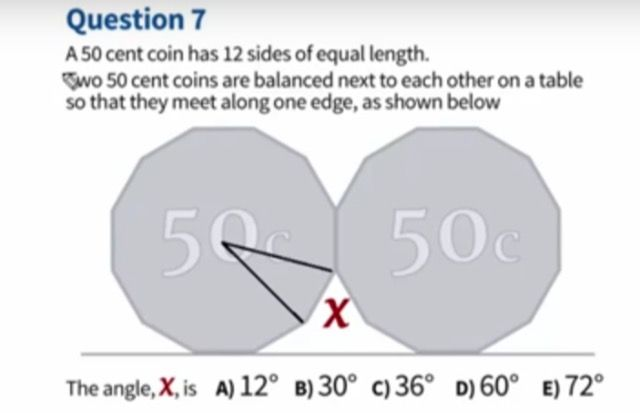# the impossible math question

Discussion in 'Off Topic' started by WOLFIE, Nov 4, 2015.

1.Student in australia apparently struggled with this deeming it impossible. Can you works it out

#1
2. 60

#2
3. 360 divide by 12 = 30. Answer is B.

#3
4. Wrong. Good try. Have a cookie. You solved for the interior angle of the 12 sided figure. Not angle x.

#4
5. 360/12*2 since there are 2 coins

#5
6. The diagram isn't really clear though, it's asking for the angle between the 2 12 sided polygons. I made same mistake at first glance.

#6
7. Op, do your own homework

#7
8. Aussies.... Smh

#8
9. You first need to find the angles of the triangle in the first figure.

The interior angle is 30 because 360/12 is 30.

Next it is a triangle with 2 equal sides, so 180 equals 30 plus 2x. Solving for x you get 75.

Next you make another equation. This equation is 360 equals 75 plus 75 plus 75 plus 75 plus x. Solve for x and you get x equals 60. Pretty easy.

#9
10.Bored at work so here

#10
11. Excellent work, Troll. I give you a lot of credit on this one.
Maybe, I would have went that route if the angle on the left coin wasn't marked. I was also questioning the relevance of the 2nd coin, but you explained it well.
Can I share my cookie with you? Lol

#11
12. Dk how this qn is categorized as impossible...

#12
13. Just takes some critical thinking... Not really difficult once you break it down.

#13
14. Lol, the only challenging part is figuring out which angle is x, cause they did not specify which angle is x. Otherwise, its basic math

#14
15. Yeah.. You could also just glance at the problem and realize that it is an equilateral triangle.

180/3 equals 60. But that's probably not how it's supposed to be done.

#15
16. *The impossible maths question.

#16
17. 360/12 gives external angle of the coin, x2 gives 60 which is angle x... The students who found it difficult must pretty dumb...

#17
18. That's not really the correct way to get the answer. Just convenient it works out to be.

#18
19. Sohcahtoa

#19
20. Jk

#20